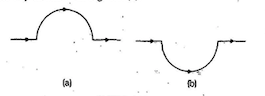Home/Class 12/Physics/

## QuestionPhysicsClass 12

A straight wire carrying a current of $$12\;A$$  is bent into a semi-circular arc of radius $$\text{2.0 cm}$$  as shown in Fig.$$(a).$$ Consider the magnetic field $$B$$  at the Centre of the arc.
In what way the contribution to $$B$$  from the semicircle differs from that of a circular loop and in what way does it resemble?For all segments of the semicircular arc, $$\mathit{dl}\times r$$ are all parallel to each other $$($$into the plane of the paper$$).$$ All such contributions add up in magnitude. Hence direction of $$B$$ for a semicircular arc is given by the right-hand rule and magnitude is half that of a circular loop.
Thus $$B=\frac{\mu_0I}{2R}$$
Here,
$$R=$$ radius $$=2\ cm\ =\ 0.02\ m$$
$$I\ =\$$current $$=\ 12\ A$$
$$\mu_0=4\pi\times10^{-7}\ Tm/A$$
$$\Rightarrow B=\frac{4\pi\times10^{-7}\times12}{2\times0.02}=1.9\times 10^{-4}\; \text T$$ normal to the plane of the paper going into it.